The Stokes Polarization Parameters

Excerpt from Field Guide to Polarization

The most serious limitation to the Poincaré sphere and the polarization ellipse are (1) the polarization ellipse is an instantaneous representation of polarized light, and (2) neither the rotation angle ψ nor the ellipticity angle χ is directly measurable. In order to overcome these limitations it is necessary to determine the measurables of the polarized field. This can be done by taking a time average of the polarization ellipse: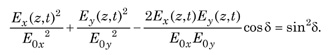The time average Ei(z,t)Ej(Z,t) is defined by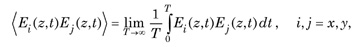where T is total averaging time. Applying the time average definition to the polarization ellipse then yields the following equation: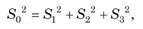where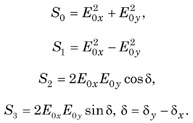The quantities S0, S1, S2, and S3 are the observables of the polarized field. They were introduced by Stokes (1852) and are called the Stokes polarization parameters.

The first Stokes parameter S0 describes the total intensity of the optical beam; the second parameter S1 describes the preponderance of LHP light over LVP light; the third parameter S2 describes the preponderance of L+45P light over L-45P light and, finally, S3 describes the preponderance of RCP light over LCP light.

The Stokes parameters can be expressed in complex notation (in order to bypass formally the time integration) by suppressing the propagator and writing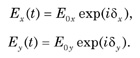The Stokes parameters are then defined in complex notation by the following equations.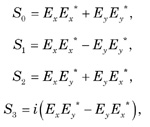where i = √−1 and * represents the complex conjugate.

It is convenient to arrange the Stokes parameters as a column matrix, which is referred to as the Stokes vector for elliptically polarized light: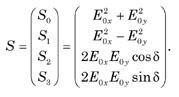Stokes Parameter Relations

The Stokes vectors for the degenerate polarization states are readily found using the previous definitions and equations: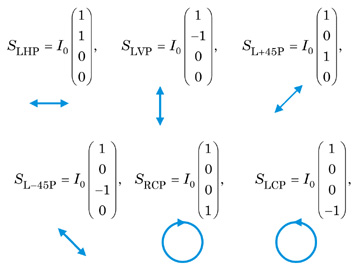where I0 is the intensity and is very often normalized to unity.

The Stokes parameters can be shown to be related to the orientation and ellipticity angles, ψ and χ, associated with the Poincaré sphere as follows: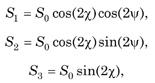and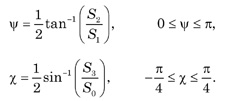Citation:

E. Collett, Field Guide to Polarization, SPIE Press, Bellingham, WA (2005).